# Developing Skills in QBASIC (Conditional Statements)

## Fill in the blanks

#### Question 1

The extension of a QBASIC file is bas.

#### Question 2

The maximum number of characters that the primary name of a QBASIC file contains is eight.

#### Question 3

QBASIC works in a Windows environment.

#### Question 4

IF statement must accomplish with THEN statement in QBASIC programming.

#### Question 5

GOTO statement develops an infinite loop in a program.

#### Question 6

A function key to execute QBASIC programs is F5.

#### Question 7

END statement indicates that the program is over.

#### Question 8

A statement which shifts the control to another line is GOTO.

#### Question 9

A command used to execute a QBASIC program is RUN.

#### Question 10

PRINT statement shows the output of a program during execution.

## Correct the mistakes in the following flowcharts and write the programs

#### Question 1

The given flowchart is to accept the length in centimeters and express it in metres (if the length exceeds 100 centimeters or more), otherwise in centimeters.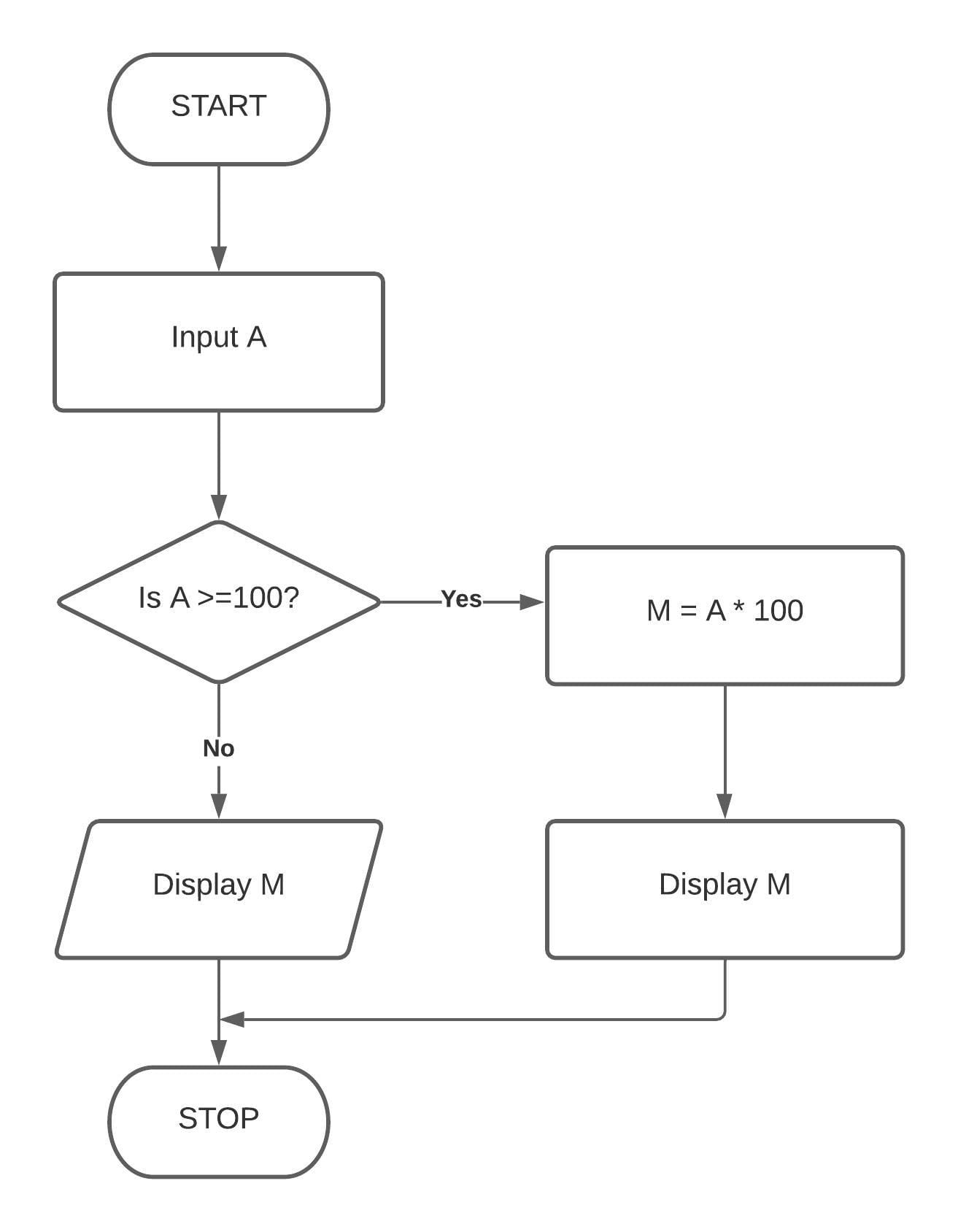FlowchartQBASIC Program

``````Cls
Input "ENTER LENGTH"; A
If A >= 100 Then
M = A * 100
Print M
Else
Print A
End If
End
``````

Output

``````ENTER LENGTH? 562
56200
``````

#### Question 2

The given flowchart is to accept one positive and one negative number. It also checks whether the sum is a positive number or a negative number.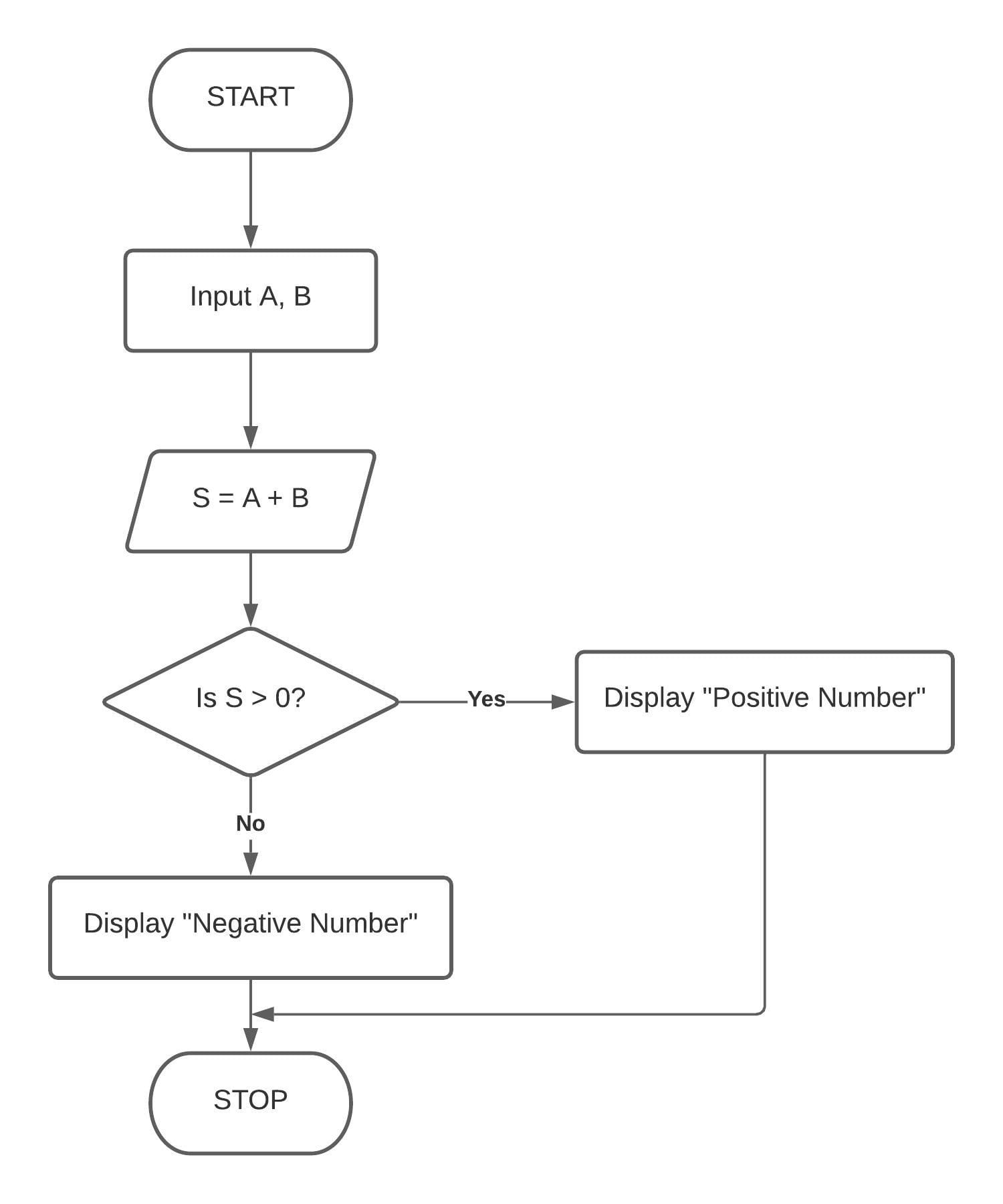Flowchart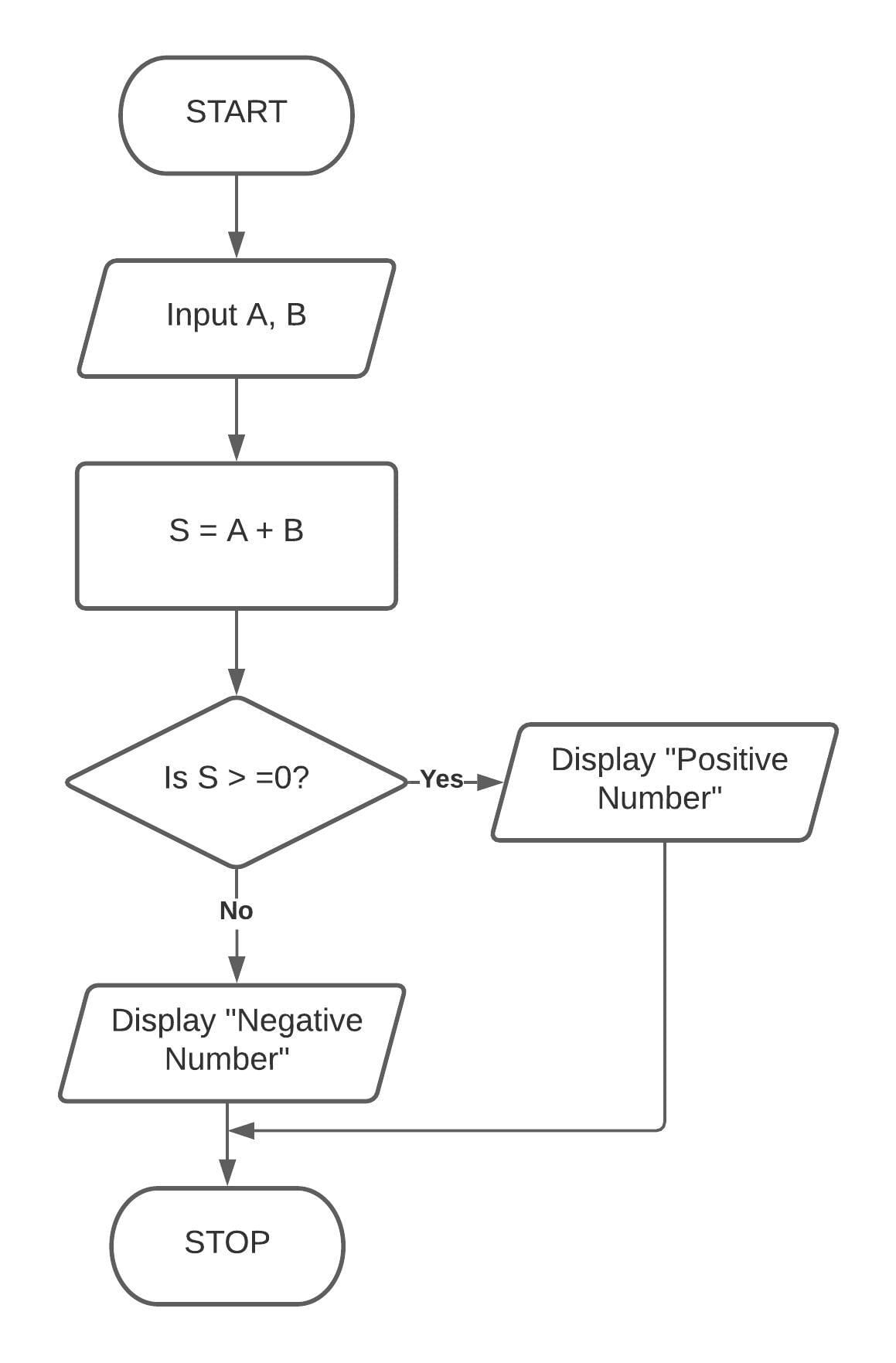QBASIC Program

``````Cls
Input "ENTER TWO NUMBERS"; A, B
Let S = A + B
If S >= 0 Then Print "POSITIVE NUMBER" Else Print "NEGATIVE NUMBER"
End
``````

Output

``````ENTER TWO NUMBERS? 40,-60
NEGATIVE NUMBER
``````

## Display the output of the following programs

#### Question 1

CLS
P = 5
START:
PRINT P
P = P + 5
IF P <= 50 THEN GOTO START:
END

The output of the program is shown below:

``````5
10
15
20
25
30
35
40
45
50
``````

#### Question 2

CLS
P = 2
Q = 1
START:
PRINT P*Q
Q = Q + 1
IF Q <=10 THEN GOTO START:
END

The output of the program is shown below:

``````2
4
6
8
10
12
14
16
18
20
``````

#### Question 3

CLS
A = 5
B = 10
START:
C = A + B
PRINT C
A = A + 1
IF A <=10 THEN GOTO START:
END

The output of the program is shown below:

``````15
16
17
18
19
20
``````

#### Question 4

CLS
C = 3
D = C*2
START:
PRINT C,D
C = C + 2
IF C <=10 THEN GOTO START:
END

The output of the program is shown below:

``````3  	  6
5 	  6
7 	  6
9 	  6
``````

#### Question 1

What is a counter? Explain with reference to a program.

Counters are numeric variables that are used to keep a record of the number of times a process is repeated. A counter is initialised with a numeric value. and it increases by 1 as the process starts repeating.

For example, suppose we want to print our name five times on the screen, then we use counter.

Program:

``````CLS
C=1
LET N\$ = "TOM"
START:
PRINT N\$
C = C + 1
IF C <=5 THEN GOTO START:
END
``````

Output:

``````TOM
TOM
TOM
TOM
TOM
``````

Here C is the counter variable and keeps on incrementing till it reaches 5.

#### Question 2

What are the different types of conditional statements used in QBASIC programming? Explain.

There are two conditional statements used in QBASIC. They are as follows:

1. IF - THEN statement: This statement checks only the 'true' part of the program and comes to an end. It is used to check a condition and then perform any task based on the given condition.

Syntax:

``````IF <condition is true> THEN <Perform the task>
``````

Example:

``````IF A > 0 THEN PRINT "A IS POSITIVE"
``````

2. IF - THEN with ELSE statement: This statement performs either of the two specified tasks based on a given condition whether it is 'true' or 'false'.

Syntax:

``````IF <condition is true> THEN <Task> ELSE <Another task>
``````

Example:

``````LET A = 5
IF A >= 0 THEN PRINT "A IS POSITIVE" ELSE PRINT "A IS NEGATIVE"
``````

3. IF - ELSEIF statement: This statement allows us to check a secondary condition if the first condition is 'False'.

Syntax:

``````IF <condition 1 is true> THEN <task>
ELSEIF <condition 2 is true> THEN <another task>
END IF
``````

Example:

``````LET A=5
IF A >= 0 THEN PRINT "A IS POSITIVE"
ELSEIF A < 0 THEN PRINT "A IS NEGATIVE"
END IF
``````

#### Question 3

An unconditional GOTO sets up an infinite loop. Explain with an example.

A GOTO statement directs the control to transfer the desired label to set up a loop. An unconditional transfer of control sets up an infinite loop, from where there is no exit.

Example:

``````CLS
A = 1
START:
PRINT A
A = A + 1
GOTO START:
END
``````

Here, after the value of A is incremented, the control transfers to the label unconditionally. So, this GOTO sets up an infinite loop.

#### Question 4

Define accumulator. Is it necessary to initialise an accumulator?

An accumulator is a numeric variable which when defined within a program, stores the data value in the memory which keeps on changing or getting updated with the counter or some other values supplied through input.

It is necessary to initialise an accumulator to avoid getting garbage value during the execution of the program.

#### Question 5

What are the different types of relational operators used along with a conditional statement in a program?

The different kinds of relational operators which are used along with a conditional statement in a program are:

1. Less than ( < )
2. More than ( > )
3. Less than or equal to ( <= )
4. Greater than or equal to ( >= )
5. Not equal to ( <> )

#### Question 6

What are the features of QBASIC as a programming language?

The features of QBASIC as a programming language are as follows:

1. It is easy to learn and is popular among beginners as the first programming language. It is specially suited for a non-specialist programmer.
2. It is available for all systems of computers such as personal computers, mini computers etc.
3. It is a high-level language that uses a compiler as a translator.
4. It is also suitable for solving problems.
5. It has graphics and sound generation capabilities.
6. Debugging can be done easily.

#### Question 7

Can you write a new program without saving the previous program? Comment.

Yes, we can write a new program without saving the previous program.

When we try to write a new program and select 'New' option from the 'File' menu, QBASIC displays a confirmation message - "Program is not saved. Save it now?" The user has a choice of 'No' in case he doesn't want to save the previous program. If the user clicks on 'No', the previous program is not saved and a new editor is opened for writing the new program.

#### Question 8

What will happen if you save two different programs with the same file name?

When we try to save the second program with the same name as another program, a message is displayed - "File already exists. Overwrite?"

The user has a choice of overwriting the previous program file or saving the new program with a different name.

## Write the syntax of the following

#### Question 1

IF - THEN

Syntax:

``````IF <condition is true> THEN <Perform the task>
``````

#### Question 2

IF - THEN - ELSE

Syntax:

``````IF <condition is true> THEN <Task> ELSE <Another task>
``````

## Solve the following programs

#### Question 1

Draw a flowchart and write a program in QBASIC to enter two numbers. Subtract the second number from the first if the first number is greater than the second number or vice-versa.

Flowchart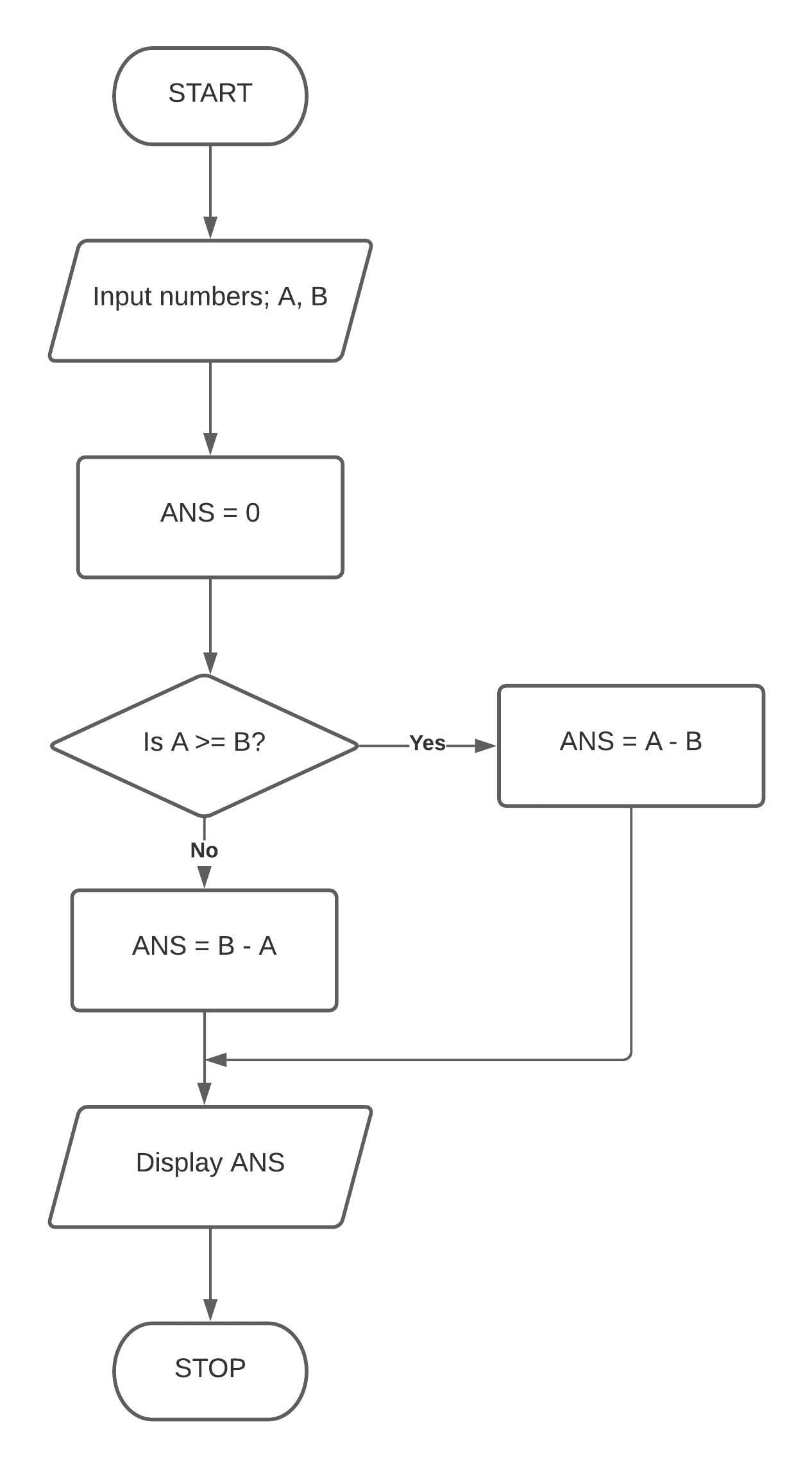QBASIC Program

``````Cls
Input "ENTER A NUMBER"; A
Input "ENTER ANOTHER NUMBER"; B
Let ANS = 0
If A > B Then ANS = A - B Else ANS = B - A
End
``````

Output

``````ENTER A NUMBER? 70
ENTER ANOTHER NUMBER? 20
``````

#### Question 2

Draw a flowchart and write a program in QBASIC to enter the numerator and denominator of a fraction. Check and print whether the fraction is proper or improper. The program will also display the difference between the numerator and the denominator accordingly.

Flowchart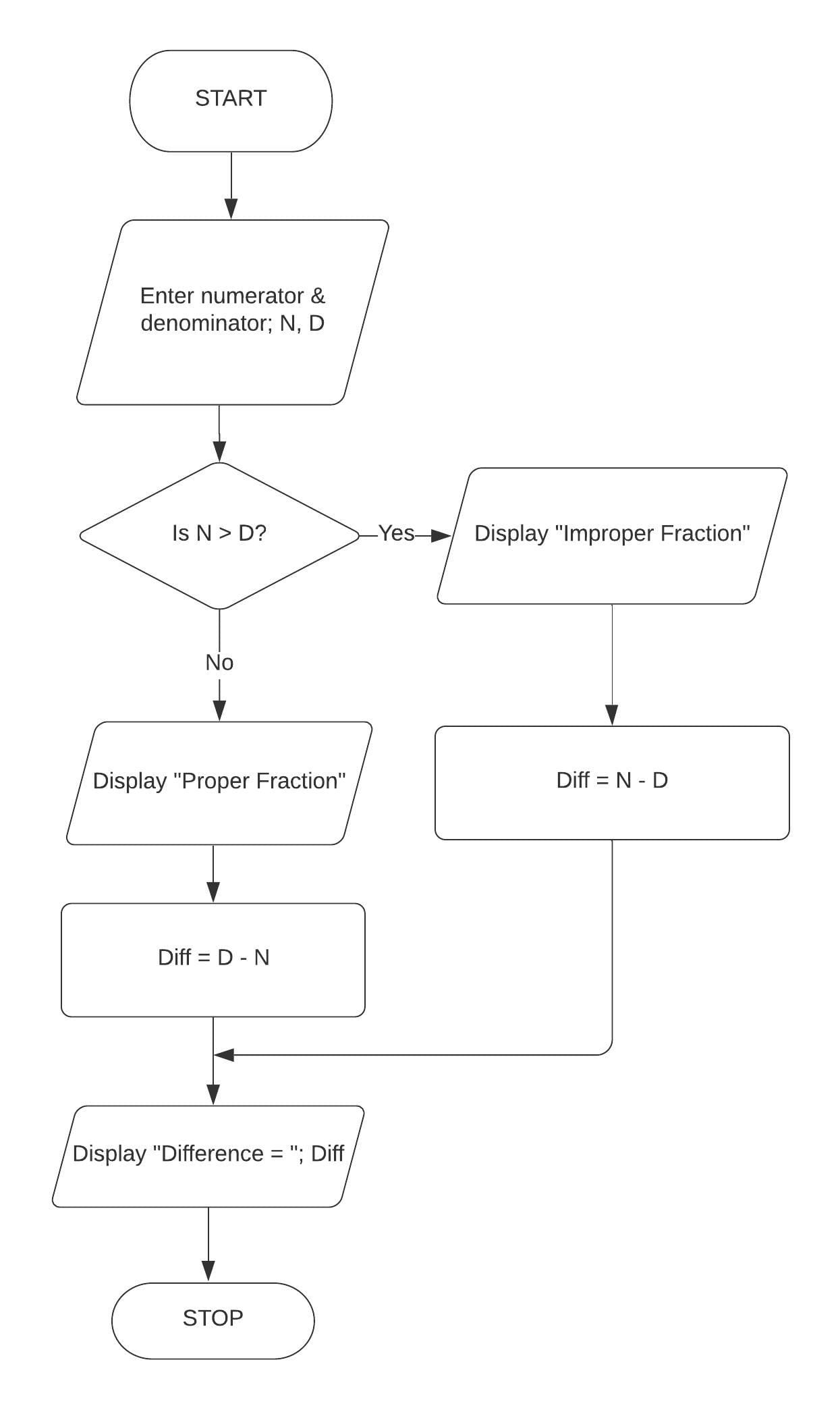QBASIC Program

``````Cls
Input "ENTER NUMERATOR"; N
Input "ENTER DENOMINATOR"; D
Let DIFF = 0
If N > D Then
Print "IMPROPER FRACTION"
Let DIFF = N - D
Else
Print "PROPER FRACTION"
DIFF = D - N
End If
Print "DIFFERENCE = "; DIFF
End
``````

Output

``````ENTER NUMERATOR? 15
ENTER DENOMINATOR? 10
IMPROPER FRACTION
DIFFERENCE = 5
``````

#### Question 3

Draw a flowchart and write a program in QBASIC to accept three angles of a triangle. The program will check whether the triangle is possible or not.

Flowchart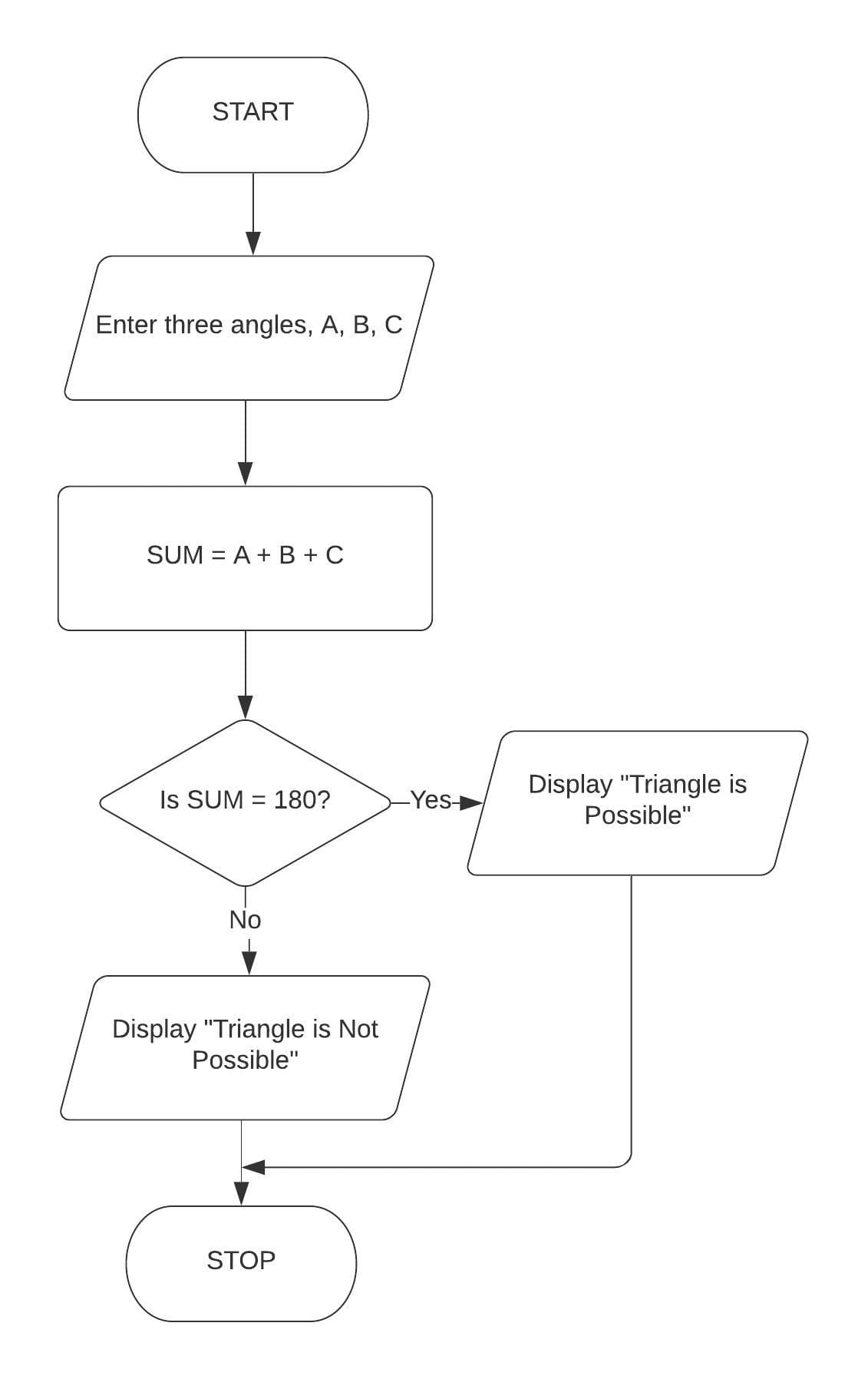QBASIC Program

``````Cls
Input "FIRST ANGLE"; A
Input "SECOND ANGLE"; B
Input "THRID ANGLE"; C
Let SUM = A + B + C
If SUM = 180 Then Print "TRIANGLE IS POSSIBLE" Else Print "TRIANGLE IS NOT POSSIBLE"
End
``````

Output

``````FIRST ANGLE? 40
SECOND ANGLE? 80
THIRD ANGLE? 30
TRIANGLE IS NOT POSSIBLE
``````

#### Question 4

Draw a flowchart and write a program in QBASIC to enter your name and the marks obtained in 10 different subjects in a term examination. Display the sum and average on the screen along with the name.

Flowchart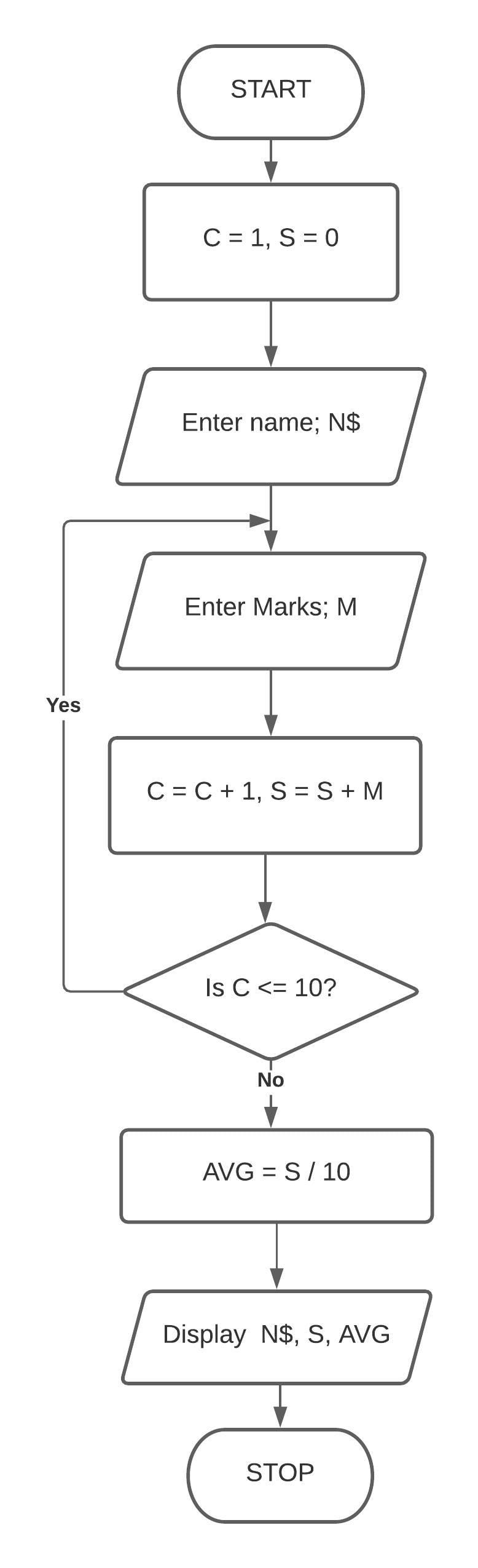QBASIC Program

``````Cls
Input "ENTER NAME"; N\$
Let C = 1
Let S = 0
START:
Input "ENTER MARKS"; M
S = S + M
C = C + 1
If C <= 10 Then GoTo START:
Print "NAME - "; N\$
Print "TOTAL = "; S
Let AVG = S / 10
Print "AVERAGE = "; AVG
End
``````

Output

``````ENTER NAME? RICHA
ENTER MARKS? 87
ENTER MARKS? 89
ENTER MARKS? 89
ENTER MARKS? 90
ENTER MARKS? 99
ENTER MARKS? 95
ENTER MARKS? 98
ENTER MARKS? 89
ENTER MARKS? 85
ENTER MARKS? 89
NAME - RICHA
TOTAL = 910
AVERAGE = 91
``````

#### Question 5a

Draw a flowchart and write a program in QBASIC to display the first 10 terms of the series:
11, 17, 23, .........

Flowchart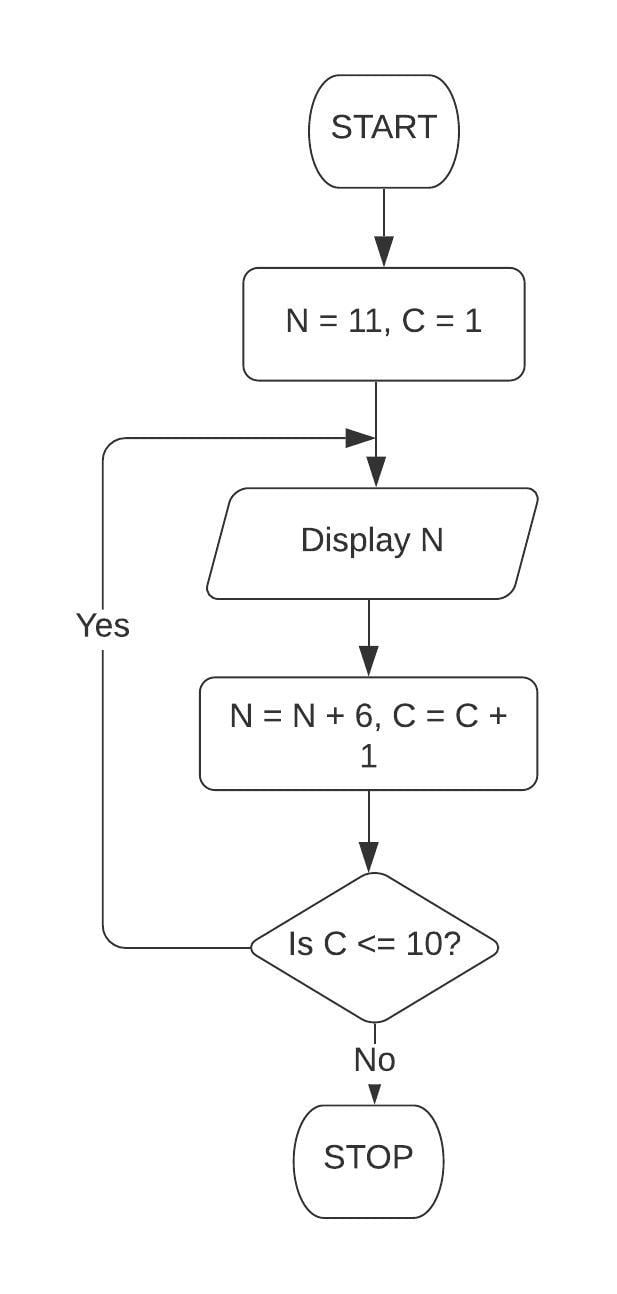QBASIC Program

``````Cls
Let N = 11
Let C = 1
START:
Print N
C = C + 1
N = N + 6
If C <= 10 Then GoTo START:
End
``````

Output

``````11
17
23
29
35
41
47
53
59
65
``````

#### Question 5b

Draw a flowchart and write a program in QBASIC to display the first 10 terms of the series:
144, 121, 100, .........

Flowchart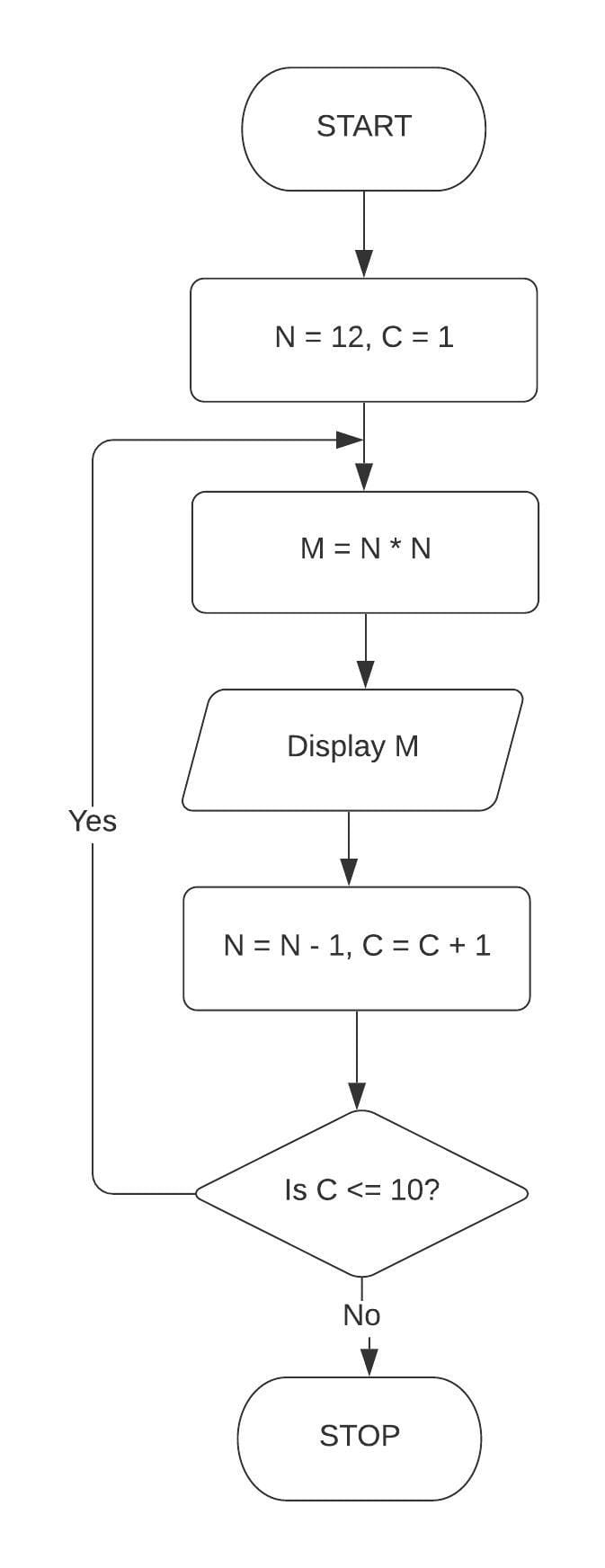QBASIC Program

``````Cls
Let N = 12
Let C = 1
START:
Let M = N * N
Print M
C = C + 1
N = N - 1
If C <= 10 Then GoTo START:
End
``````

Output

``````144
121
100
81
64
49
36
25
16
9
``````

#### Question 6a

Draw a flowchart and write a program in QBASIC to print the first 10 terms of the series:
S = 7 + 11 + 15 + 19 + .........

Flowchart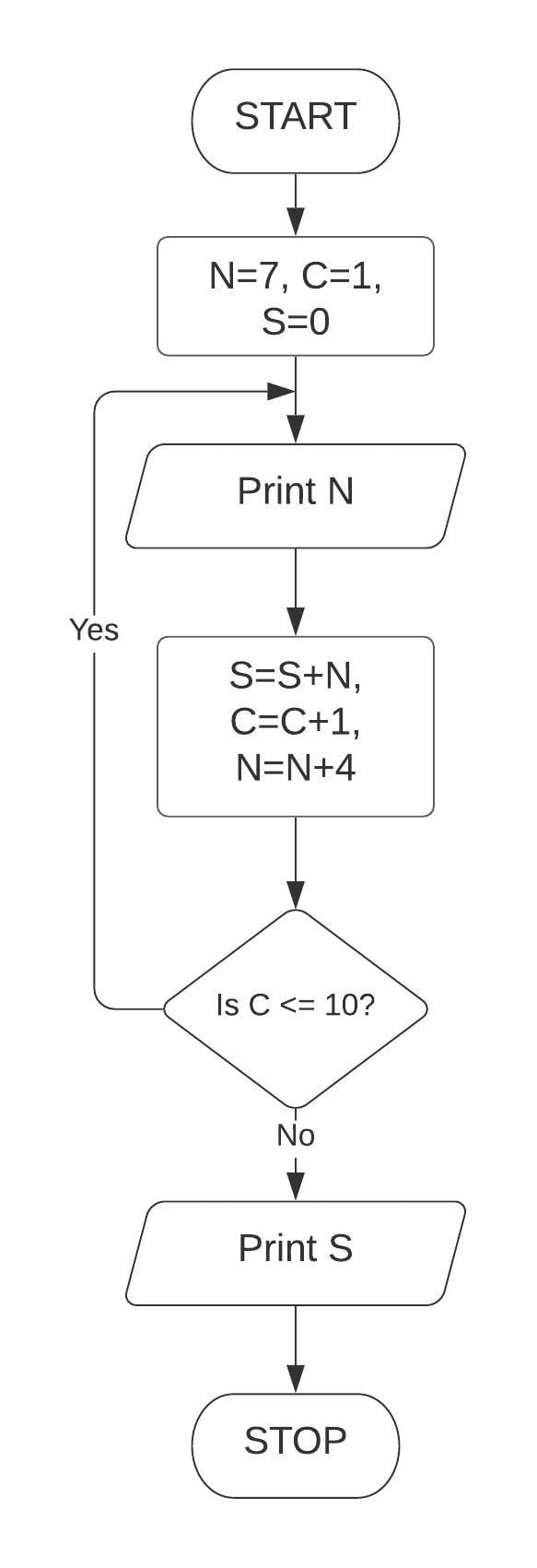QBASIC Program

``````Cls
Let N = 7
Let C = 1
Let S = 0
START:
Print N
S = S + N
C = C + 1
N = N + 4
If C <= 10 Then GoTo START:
Print "Sum = "; S
End
``````

Output

``````7
11
15
19
23
27
31
35
39
43
Sum = 250
``````

#### Question 6b

Draw a flowchart and write a program in QBASIC to print the first 10 terms of the series:
S = 3 + 6 + 12 + 24 + .........

Flowchart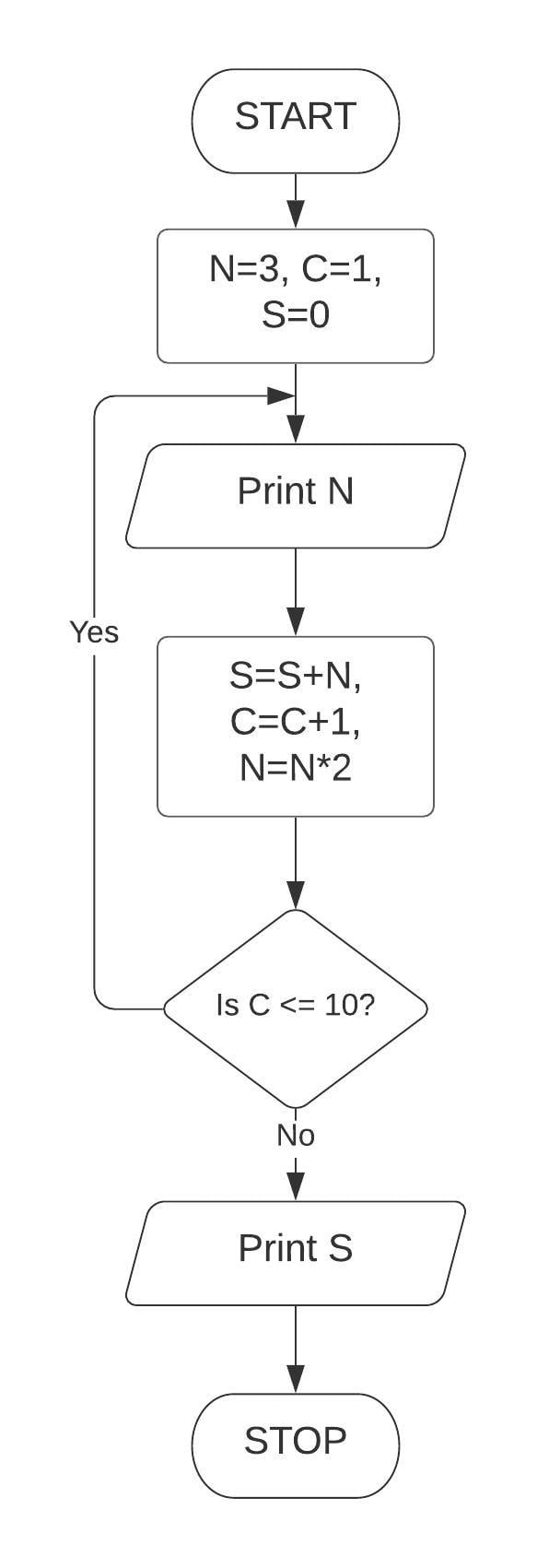QBASIC Program

``````Cls
Let N = 3
Let C = 1
Let S = 0
START:
Print N
S = S + N
C = C + 1
N = N * 2
If C <= 10 Then GoTo START:
Print "SUM = "; S
End
``````

Output

``````3
6
12
24
48
96
192
384
768
1536
SUM = 3069
``````